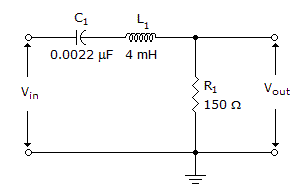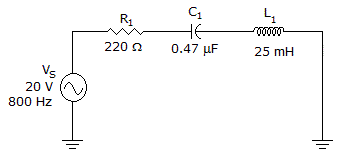# Electronics - RLC Circuits and Resonance

### Exercise :: RLC Circuits and Resonance - General Questions

36.What is the resonant frequency in the given circuit?

 A. 6.5 kHz B. 53.7 kHz C. 107.4 kHz D. 18.1 MHz

Answer: Option B

Explanation:

No answer description available for this question. Let us discuss.

37.What is the impedance of the circuit in the given circuit?

 A. 125.7B. 297.6C. 370.1D. 423.3Answer: Option C

Explanation:

No answer description available for this question. Let us discuss.

38.

What is the approximate phase angle in a series RLC circuit when VC = 117 V, VR = 14.5 V, and VL = 3.3 V?

 A. –45.0 degrees B. –82.7 degrees C. –90.0 degrees D. –172.7 degrees

Answer: Option B

Explanation:

No answer description available for this question. Let us discuss.

39.

Which statement best describes reactance in a series RLC circuit?

 A. Capacitive reactance is always dominant. B. Inductive reactance is always dominant. C. Resistance is always dominant. D. The larger of the two reactances is dominant.

Answer: Option D

Explanation:

No answer description available for this question. Let us discuss.

40.

What is the band pass (F1 – F2) of an RLC filter that resonates at 150 kHz and has a coil Q of 30?

 A. 100.0 kHz to 155.0 kHz B. 147.5 kHz to 152.5 kHz C. 295.5 kHz to 4500 kHz D. 149,970 Hz to 150,030 Hz

Answer: Option B

Explanation:

No answer description available for this question. Let us discuss.

#### Current Affairs 2021

Interview Questions and Answers